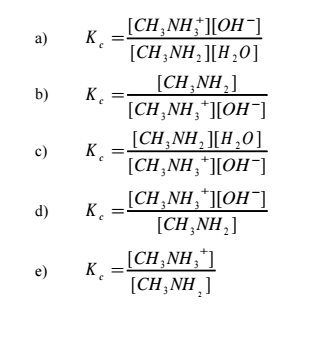Chemistry Practice Problems Equilibrium Expressions Practice Problems Solution: Methylamine is a weak base in water according to t...

🤓 Based on our data, we think this question is relevant for Professor Brown's class at TAMU.

# Solution: Methylamine is a weak base in water according to the equilibriumCH3NH2(aq) + H2O(l) ⇌ CH3NH3+(aq) + OH−(aq)What is the correct expression for the equilibrium constant?

###### Problem

Methylamine is a weak base in water according to the equilibrium

CH3NH2(aq) + H2O(l) ⇌ CH3NH3+(aq) + OH(aq)

What is the correct expression for the equilibrium constant?Equilibrium Expressions

Equilibrium Expressions

#### Q. Match each chemical equation below with the correct equilibrium constant expression.Chemical Equation                                           Equili...

Solved • Fri Mar 20 2020 17:20:58 GMT-0400 (EDT)

Equilibrium Expressions

#### Q. The temperature dependence of the equilibrium constant (Kp) is illustrated for the following reaction:NH4ClO4(s) → NH3(g) + HClO4(g)Note: the points a...

Solved • Mon Mar 16 2020 19:15:36 GMT-0400 (EDT)

Equilibrium Expressions

#### Q. Consider the reaction: 2A + B ⇌ A 2B. If the equilibrium constant, Kc, = 12.6 at a certain temp and if the reverse rate constant (kr) = 5.1 x 10 -2 s ...

Solved • Mon Apr 18 2016 17:44:59 GMT-0400 (EDT)

Equilibrium Expressions

#### Q. When glucose, a sugar, reacts fully with oxygen,carbon dioxide and water are produced according to the equation:C6H12O6(s) + 6 O2(g) ⟺ 6 CO2(g) + 6 H2...

Solved • Fri Mar 11 2016 16:26:46 GMT-0500 (EST)